### Mathematics JSS2 Second Term Final Assessment

Assessment

Solve the following inequalities

6 < 1 – 2x

1. X <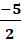2. X <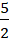3. X <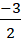4. X <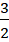5x + 2 > 11x – 10

1. X <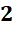2. X <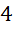3. X <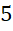4. X <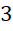3x < 9

1. X <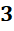2. X ≤3. X <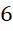4. X ≤2x – 3 ≤ x +.... View More